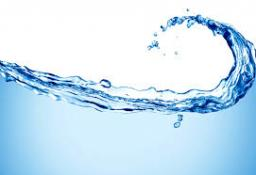# Liters 3107

Find how many liters of water at 80 °C and 20 °C need to mix to get 30 liters at 60 °C.

a =  20
b =  10

### Step-by-step explanation:

80a+20b = 30·60
a+b = 30

80·a+20·b = 30·60
a+b = 30

80a+20b = 1800
a+b = 30

Row 2 - 1/80 · Row 1 → Row 2
80a+20b = 1800
0.75b = 7.5

b = 7.5/0.75 = 10
a = 1800-20b/80 = 1800-20 · 10/80 = 20

a = 20
b = 10

Our linear equations calculator calculates it.Did you find an error or inaccuracy? Feel free to write us. Thank you!

Tips for related online calculators
Do you have a system of equations and looking for calculator system of linear equations?
Tip: Our volume units converter will help you convert volume units.